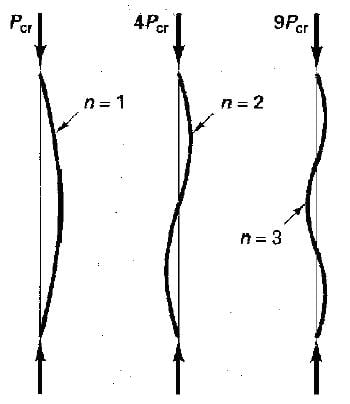# Buckling plate

When buckling plate of both ends fixed, the deflection and the mode shape of the plate can be found from the equation: y=M(1-cos mx)/P. With this equation, the cross sectional area and the young's modulus of the plate is usually unchange.
However, if the flexural rigidity of the plate is divided into three segments such that the middle segment have a higher flexural rigidity than the other two segments, it is found that the equation: y=M(1-cos mx)/P seems not fit this situation.
Would you know how to calculate the deflection and the mode shape in this case, please? Thank you in advance.

Generally you can estimate the mode shapes by creating a stiffness matrix (a series of equations at each degree of freedom) and then modifying the stiffness matrix by making it a function of the axial load. This modified matrix is called a geometric stiffness matrix.

You then have an Eigen value problem which you solve for all the buckling modes. This is what a Finite Element computer program would be essentially doing.

Now maybe the above is more technically gobbly-gook than you wanted and maybe there is a more specifically simplified solution. But hopefully you can start to do some research and come with an approach for this.

paisiello 2, thanks for your reply.
Pardon me that I may have a typing mistake of mode shape in my post. I am not doing vibration analysis, so don't need to calculate mode shape, right? (Please correct me if I am wrong).
If i just need to know the deflection equation of the three segment plate under axial loading, should I calculate stiffness matrix? If you have some hints for the calculation of the deflection equation, please let me know. Thank you!

Well, you did ask how to calculate the mode shape in your OP.

Buckling mode shapes are technically not the same thing as vibrational mode shapes. The former are the different ways in which a structure will become unstable while the latter are the different ways that a structure will move dynamically, although they both might be mathematically the same in some simple cases. For example, here are the first three mode shapes for buckling of a pin-pinned column:These would be the same as the first three vibrational mode shapes.

Regardless, I think the approach I described previously is the easiest and the most general. There may be an exact mathematical solution but I think it would be more complicated. You might be able to find where someone has gone through this and posted a solution already.

Thank you! I am now reading some relevant books and articles. Hope I can solve it soon.